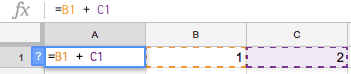# xcell

xcell is a tiny, open source (MIT) library for building reactive, spreadsheet-like calculations in JavaScript.

Spreadsheets are cool—if we say that A1 is the sum of B1 and C1, the spreadsheet will automatically update whenever we change the dependent cells.This usually doesn't happen in our programs.

For example in JavaScript:

``````var b = 1, c = 2

var a = b + c // a is 3 now

b = 42

alert("a is now:  " + a) // it is still 3 :(``````

our variable a does not automatically change if we change b. It will be equal to 3 until we imperatively change it something else.

xcell allows us to write programs that work like spreadsheets.

Here is how:

``````function add(x, y) {
return x + y
}

var b = xcell(1), c = xcell(2)

var a = xcell([b, c], add)

b.value = 42

alert(a.value) // a is 44 \o/``````

`xcell` is a function that returns an object that holds always updated value, just like a spreadsheet cell.

When we create our "cells" we tell them to either be independent:

``var b = xcell(1)``

or to depend on other cells and update its value when necessary using a provided function:

``var a = xcell([b, c], add)``

The cells emit `change` event whenever they change, so we can observe them and update our UI:

``````a.on('change', function handleChange(sender) {
document.getElementById("my-cell").value = sender.value
})``````

Here are a few examples of how xcell can be used:

The source code is on github.